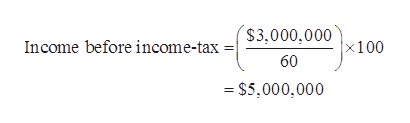Little Books, Inc. recently reported \$3 million of net income. Its EBIT was \$6 million, and its tax rate was 40%. What was its interest expense? (Hint: write out the headings for an income statement and then fill in the known values. Then divide \$3 million net income by (1-T) to find pre-tax income. The difference between EBIT and taxable income must be the interest expense. Use this same procedure to work some of the other problems.)

Question

Little Books, Inc. recently reported \$3 million of net income. Its EBIT was \$6 million, and its tax rate was 40%. What was its interest expense? (Hint: write out the headings for an income statement and then fill in the known values. Then divide \$3 million net income by (1-T) to find pre-tax income. The difference between EBIT and taxable income must be the interest expense. Use this same procedure to work some of the other problems.)

Step 1

Calculation of interest expenses:

Given, tax rate is 40% then, Net income will be 60%

...help_outlineImage Transcriptionclose\$3,000.000100 Income before income-tax 60 = \$5,000,000 fullscreen

Want to see the full answer?

See Solution

Want to see this answer and more?

Our solutions are written by experts, many with advanced degrees, and available 24/7

See Solution
Tagged in